# Plotting¶

## Introduction¶

The plotting module allows you to make 2-dimensional and 3-dimensional plots. Presently the plots are rendered using matplotlib as a backend. It is also possible to plot 2-dimensional plots using a TextBackend if you don’t have matplotlib.

The plotting module has the following functions:

• plot: Plots 2D line plots.

• plot_parametric: Plots 2D parametric plots.

• plot_implicit: Plots 2D implicit and region plots.

• plot3d: Plots 3D plots of functions in two variables.

• plot3d_parametric_line: Plots 3D line plots, defined by a parameter.

• plot3d_parametric_surface: Plots 3D parametric surface plots.

The above functions are only for convenience and ease of use. It is possible to plot any plot by passing the corresponding Series class to Plot as argument.

## Plot Class¶

class sympy.plotting.plot.Plot(*args, **kwargs)[source]

The central class of the plotting module.

For interactive work the function plot is better suited.

This class permits the plotting of sympy expressions using numerous backends (matplotlib, textplot, the old pyglet module for sympy, Google charts api, etc).

The figure can contain an arbitrary number of plots of sympy expressions, lists of coordinates of points, etc. Plot has a private attribute _series that contains all data series to be plotted (expressions for lines or surfaces, lists of points, etc (all subclasses of BaseSeries)). Those data series are instances of classes not imported by from sympy import *.

The customization of the figure is on two levels. Global options that concern the figure as a whole (eg title, xlabel, scale, etc) and per-data series options (eg name) and aesthetics (eg. color, point shape, line type, etc.).

The difference between options and aesthetics is that an aesthetic can be a function of the coordinates (or parameters in a parametric plot). The supported values for an aesthetic are: - None (the backend uses default values) - a constant - a function of one variable (the first coordinate or parameter) - a function of two variables (the first and second coordinate or parameters) - a function of three variables (only in nonparametric 3D plots) Their implementation depends on the backend so they may not work in some backends.

If the plot is parametric and the arity of the aesthetic function permits it the aesthetic is calculated over parameters and not over coordinates. If the arity does not permit calculation over parameters the calculation is done over coordinates.

Only cartesian coordinates are supported for the moment, but you can use the parametric plots to plot in polar, spherical and cylindrical coordinates.

The arguments for the constructor Plot must be subclasses of BaseSeries.

Any global option can be specified as a keyword argument.

The global options for a figure are:

• title : str

• xlabel : str

• ylabel : str

• legend : bool

• xscale : {‘linear’, ‘log’}

• yscale : {‘linear’, ‘log’}

• axis : bool

• axis_center : tuple of two floats or {‘center’, ‘auto’}

• xlim : tuple of two floats

• ylim : tuple of two floats

• aspect_ratio : tuple of two floats or {‘auto’}

• autoscale : bool

• margin : float in [0, 1]

The per data series options and aesthetics are: There are none in the base series. See below for options for subclasses.

Some data series support additional aesthetics or options:

ListSeries, LineOver1DRangeSeries, Parametric2DLineSeries, Parametric3DLineSeries support the following:

Aesthetics:

• line_color : function which returns a float.

options:

• label : str

• steps : bool

• integers_only : bool

SurfaceOver2DRangeSeries, ParametricSurfaceSeries support the following:

aesthetics:

• surface_color : function which returns a float.

append(arg)[source]

Adds an element from a plot’s series to an existing plot.

Examples

Consider two Plot objects, p1 and p2. To add the second plot’s first series object to the first, use the append method, like so:

>>> from sympy import symbols
>>> from sympy.plotting import plot
>>> x = symbols('x')
>>> p1 = plot(x*x, show=False)
>>> p2 = plot(x, show=False)
>>> p1.append(p2)
>>> p1
Plot object containing:
: cartesian line: x**2 for x over (-10.0, 10.0)
: cartesian line: x for x over (-10.0, 10.0)
>>> p1.show()


(png, hires.png, pdf)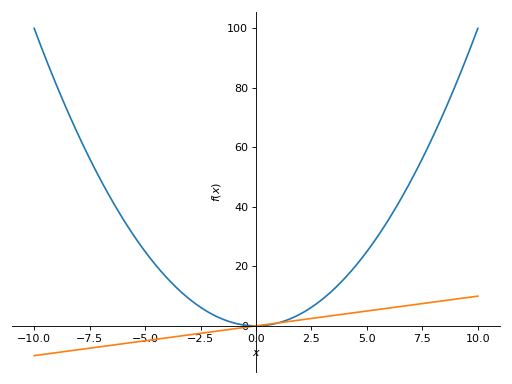extend(arg)[source]

Adds all series from another plot.

Examples

Consider two Plot objects, p1 and p2. To add the second plot to the first, use the extend method, like so:

>>> from sympy import symbols
>>> from sympy.plotting import plot
>>> x = symbols('x')
>>> p1 = plot(x**2, show=False)
>>> p2 = plot(x, -x, show=False)
>>> p1.extend(p2)
>>> p1
Plot object containing:
: cartesian line: x**2 for x over (-10.0, 10.0)
: cartesian line: x for x over (-10.0, 10.0)
: cartesian line: -x for x over (-10.0, 10.0)
>>> p1.show()


(png, hires.png, pdf)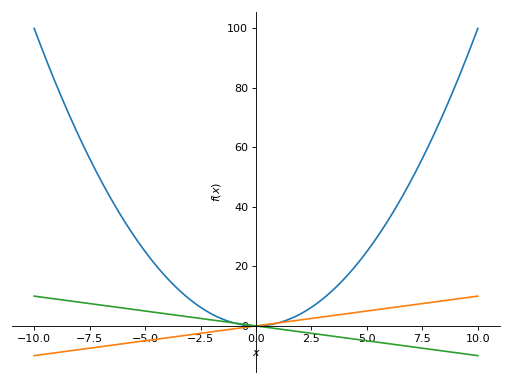## Plotting Function Reference¶

sympy.plotting.plot.plot(*args, **kwargs)[source]

Plots a function of a single variable and returns an instance of the Plot class (also, see the description of the show keyword argument below).

The plotting uses an adaptive algorithm which samples recursively to accurately plot the plot. The adaptive algorithm uses a random point near the midpoint of two points that has to be further sampled. Hence the same plots can appear slightly different.

Usage

Single Plot

plot(expr, range, **kwargs)

If the range is not specified, then a default range of (-10, 10) is used.

Multiple plots with same range.

plot(expr1, expr2, ..., range, **kwargs)

If the range is not specified, then a default range of (-10, 10) is used.

Multiple plots with different ranges.

plot((expr1, range), (expr2, range), ..., **kwargs)

Range has to be specified for every expression.

Default range may change in the future if a more advanced default range detection algorithm is implemented.

Arguments

expr : Expression representing the function of single variable

range: (x, 0, 5), A 3-tuple denoting the range of the free variable.

Keyword Arguments

Arguments for plot function:

show: Boolean. The default value is set to True. Set show to False and the function will not display the plot. The returned instance of the Plot class can then be used to save or display the plot by calling the save() and show() methods respectively.

Arguments for LineOver1DRangeSeries class:

adaptive: Boolean. The default value is set to True. Set adaptive to False and specify nb_of_points if uniform sampling is required.

depth: int Recursion depth of the adaptive algorithm. A depth of value n samples a maximum of $$2^{n}$$ points.

nb_of_points: int. Used when the adaptive is set to False. The function is uniformly sampled at nb_of_points number of points.

Aesthetics options:

line_color: float. Specifies the color for the plot. See Plot to see how to set color for the plots.

If there are multiple plots, then the same series series are applied to all the plots. If you want to set these options separately, you can index the Plot object returned and set it.

Arguments for Plot class:

title : str. Title of the plot. It is set to the latex representation of the expression, if the plot has only one expression.

xlabel : str. Label for the x-axis.

ylabel : str. Label for the y-axis.

xscale: {‘linear’, ‘log’} Sets the scaling of the x-axis.

yscale: {‘linear’, ‘log’} Sets the scaling if the y-axis.

axis_center: tuple of two floats denoting the coordinates of the center or {‘center’, ‘auto’}

xlim : tuple of two floats, denoting the x-axis limits.

ylim : tuple of two floats, denoting the y-axis limits.

annotations: list. A list of dictionaries specifying the type of annotation required. The keys in the dictionary should be equivalent to the arguments of the matplotlib’s annotate() function.

markers: list. A list of dictionaries specifying the type the markers required. The keys in the dictionary should be equivalent to the arguments of the matplotlib’s plot() function along with the marker related keyworded arguments.

rectangles: list. A list of dictionaries specifying the dimensions of the rectangles to be plotted. The keys in the dictionary should be equivalent to the arguments of the matplotlib’s patches.Rectangle class.

fill: dict. A dictionary specifying the type of color filling required in the plot. The keys in the dictionary should be equivalent to the arguments of the matplotlib’s fill_between() function.

Examples

>>> from sympy import symbols
>>> from sympy.plotting import plot
>>> x = symbols('x')


Single Plot

>>> plot(x**2, (x, -5, 5))
Plot object containing:
: cartesian line: x**2 for x over (-5.0, 5.0)


(png, hires.png, pdf)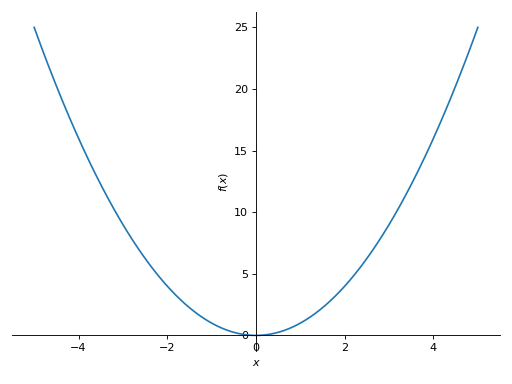Multiple plots with single range.

>>> plot(x, x**2, x**3, (x, -5, 5))
Plot object containing:
: cartesian line: x for x over (-5.0, 5.0)
: cartesian line: x**2 for x over (-5.0, 5.0)
: cartesian line: x**3 for x over (-5.0, 5.0)


(png, hires.png, pdf)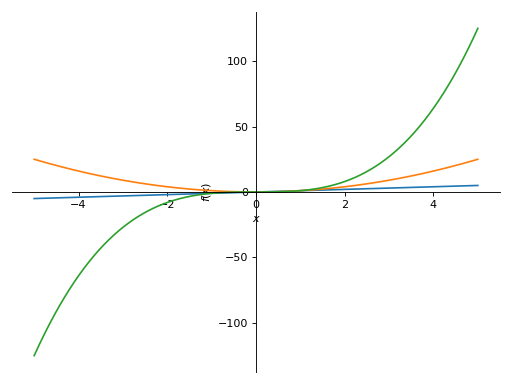Multiple plots with different ranges.

>>> plot((x**2, (x, -6, 6)), (x, (x, -5, 5)))
Plot object containing:
: cartesian line: x**2 for x over (-6.0, 6.0)
: cartesian line: x for x over (-5.0, 5.0)


(png, hires.png, pdf)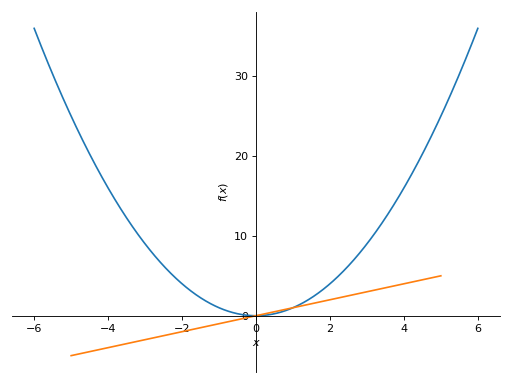>>> plot(x**2, adaptive=False, nb_of_points=400)
Plot object containing:
: cartesian line: x**2 for x over (-10.0, 10.0)


(png, hires.png, pdf)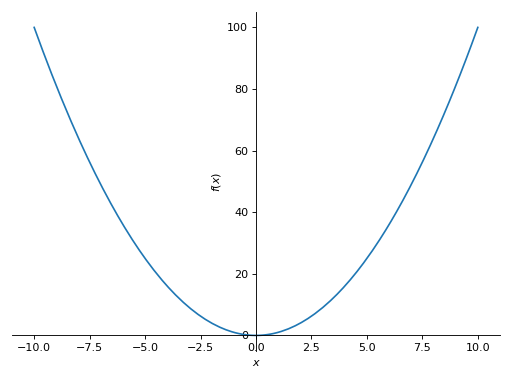sympy.plotting.plot.plot_parametric(*args, **kwargs)[source]

Plots a 2D parametric plot.

The plotting uses an adaptive algorithm which samples recursively to accurately plot the plot. The adaptive algorithm uses a random point near the midpoint of two points that has to be further sampled. Hence the same plots can appear slightly different.

Usage

Single plot.

plot_parametric(expr_x, expr_y, range, **kwargs)

If the range is not specified, then a default range of (-10, 10) is used.

Multiple plots with same range.

plot_parametric((expr1_x, expr1_y), (expr2_x, expr2_y), range, **kwargs)

If the range is not specified, then a default range of (-10, 10) is used.

Multiple plots with different ranges.

plot_parametric((expr_x, expr_y, range), ..., **kwargs)

Range has to be specified for every expression.

Default range may change in the future if a more advanced default range detection algorithm is implemented.

Arguments

expr_x : Expression representing the function along x.

expr_y : Expression representing the function along y.

range: (u, 0, 5), A 3-tuple denoting the range of the parameter variable.

Keyword Arguments

Arguments for Parametric2DLineSeries class:

adaptive: Boolean. The default value is set to True. Set adaptive to False and specify nb_of_points if uniform sampling is required.

depth: int Recursion depth of the adaptive algorithm. A depth of value n samples a maximum of $$2^{n}$$ points.

nb_of_points: int. Used when the adaptive is set to False. The function is uniformly sampled at nb_of_points number of points.

Aesthetics

line_color: function which returns a float. Specifies the color for the plot. See sympy.plotting.Plot for more details.

If there are multiple plots, then the same Series arguments are applied to all the plots. If you want to set these options separately, you can index the returned Plot object and set it.

Arguments for Plot class:

xlabel : str. Label for the x-axis.

ylabel : str. Label for the y-axis.

xscale: {‘linear’, ‘log’} Sets the scaling of the x-axis.

yscale: {‘linear’, ‘log’} Sets the scaling if the y-axis.

axis_center: tuple of two floats denoting the coordinates of the center or {‘center’, ‘auto’}

xlim : tuple of two floats, denoting the x-axis limits.

ylim : tuple of two floats, denoting the y-axis limits.

Examples

>>> from sympy import symbols, cos, sin
>>> from sympy.plotting import plot_parametric
>>> u = symbols('u')


Single Parametric plot

>>> plot_parametric(cos(u), sin(u), (u, -5, 5))
Plot object containing:
: parametric cartesian line: (cos(u), sin(u)) for u over (-5.0, 5.0)


(png, hires.png, pdf)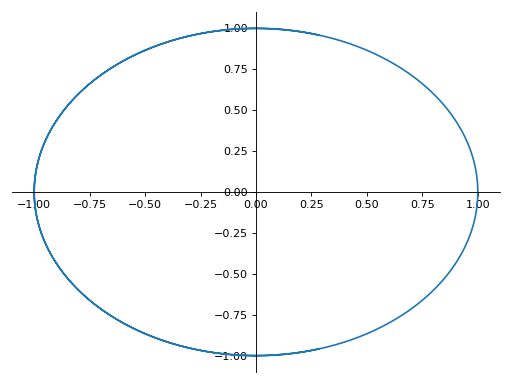Multiple parametric plot with single range.

>>> plot_parametric((cos(u), sin(u)), (u, cos(u)))
Plot object containing:
: parametric cartesian line: (cos(u), sin(u)) for u over (-10.0, 10.0)
: parametric cartesian line: (u, cos(u)) for u over (-10.0, 10.0)


(png, hires.png, pdf)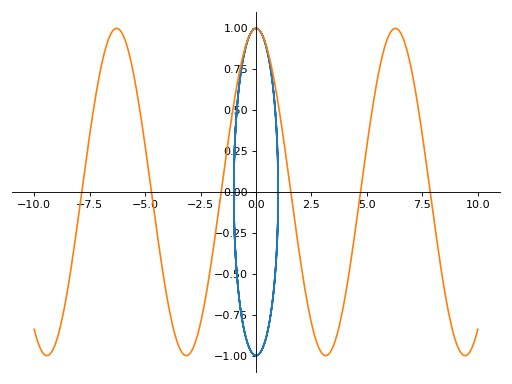Multiple parametric plots.

>>> plot_parametric((cos(u), sin(u), (u, -5, 5)),
...     (cos(u), u, (u, -5, 5)))
Plot object containing:
: parametric cartesian line: (cos(u), sin(u)) for u over (-5.0, 5.0)
: parametric cartesian line: (cos(u), u) for u over (-5.0, 5.0)


(png, hires.png, pdf)sympy.plotting.plot.plot3d(*args, **kwargs)[source]

Plots a 3D surface plot.

Usage

Single plot

plot3d(expr, range_x, range_y, **kwargs)

If the ranges are not specified, then a default range of (-10, 10) is used.

Multiple plot with the same range.

plot3d(expr1, expr2, range_x, range_y, **kwargs)

If the ranges are not specified, then a default range of (-10, 10) is used.

Multiple plots with different ranges.

plot3d((expr1, range_x, range_y), (expr2, range_x, range_y), ..., **kwargs)

Ranges have to be specified for every expression.

Default range may change in the future if a more advanced default range detection algorithm is implemented.

Arguments

expr : Expression representing the function along x.

range_x: (x, 0, 5), A 3-tuple denoting the range of the x variable.

range_y: (y, 0, 5), A 3-tuple denoting the range of the y

variable.

Keyword Arguments

Arguments for SurfaceOver2DRangeSeries class:

nb_of_points_x: int. The x range is sampled uniformly at nb_of_points_x of points.

nb_of_points_y: int. The y range is sampled uniformly at nb_of_points_y of points.

Aesthetics:

surface_color: Function which returns a float. Specifies the color for the surface of the plot. See sympy.plotting.Plot for more details.

If there are multiple plots, then the same series arguments are applied to all the plots. If you want to set these options separately, you can index the returned Plot object and set it.

Arguments for Plot class:

title : str. Title of the plot.

Examples

>>> from sympy import symbols
>>> from sympy.plotting import plot3d
>>> x, y = symbols('x y')


Single plot

>>> plot3d(x*y, (x, -5, 5), (y, -5, 5))
Plot object containing:
: cartesian surface: x*y for x over (-5.0, 5.0) and y over (-5.0, 5.0)


(png, hires.png, pdf)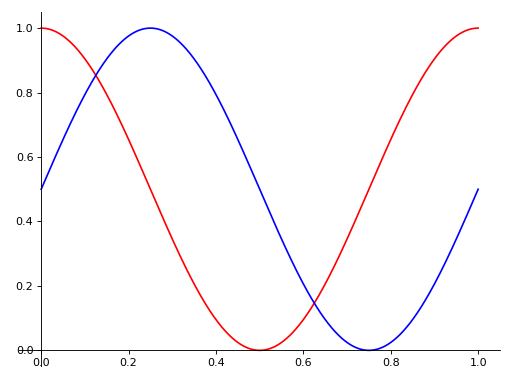Multiple plots with same range

>>> plot3d(x*y, -x*y, (x, -5, 5), (y, -5, 5))
Plot object containing:
: cartesian surface: x*y for x over (-5.0, 5.0) and y over (-5.0, 5.0)
: cartesian surface: -x*y for x over (-5.0, 5.0) and y over (-5.0, 5.0)


(png, hires.png, pdf)Multiple plots with different ranges.

>>> plot3d((x**2 + y**2, (x, -5, 5), (y, -5, 5)),
...     (x*y, (x, -3, 3), (y, -3, 3)))
Plot object containing:
: cartesian surface: x**2 + y**2 for x over (-5.0, 5.0) and y over (-5.0, 5.0)
: cartesian surface: x*y for x over (-3.0, 3.0) and y over (-3.0, 3.0)


(png, hires.png, pdf)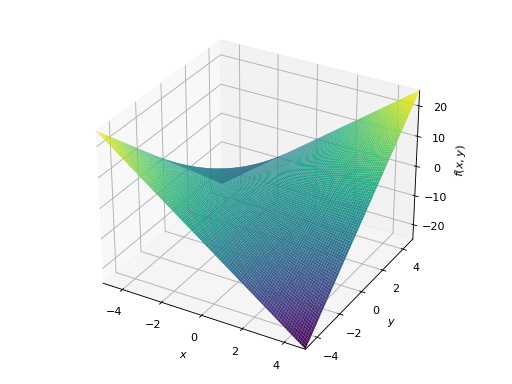sympy.plotting.plot.plot3d_parametric_line(*args, **kwargs)[source]

Plots a 3D parametric line plot.

Usage

Single plot:

plot3d_parametric_line(expr_x, expr_y, expr_z, range, **kwargs)

If the range is not specified, then a default range of (-10, 10) is used.

Multiple plots.

plot3d_parametric_line((expr_x, expr_y, expr_z, range), ..., **kwargs)

Ranges have to be specified for every expression.

Default range may change in the future if a more advanced default range detection algorithm is implemented.

Arguments

expr_x : Expression representing the function along x.

expr_y : Expression representing the function along y.

expr_z : Expression representing the function along z.

range: (u, 0, 5), A 3-tuple denoting the range of the parameter variable.

Keyword Arguments

Arguments for Parametric3DLineSeries class.

nb_of_points: The range is uniformly sampled at nb_of_points number of points.

Aesthetics:

line_color: function which returns a float. Specifies the color for the plot. See sympy.plotting.Plot for more details.

If there are multiple plots, then the same series arguments are applied to all the plots. If you want to set these options separately, you can index the returned Plot object and set it.

Arguments for Plot class.

title : str. Title of the plot.

Examples

>>> from sympy import symbols, cos, sin
>>> from sympy.plotting import plot3d_parametric_line
>>> u = symbols('u')


Single plot.

>>> plot3d_parametric_line(cos(u), sin(u), u, (u, -5, 5))
Plot object containing:
: 3D parametric cartesian line: (cos(u), sin(u), u) for u over (-5.0, 5.0)


(png, hires.png, pdf)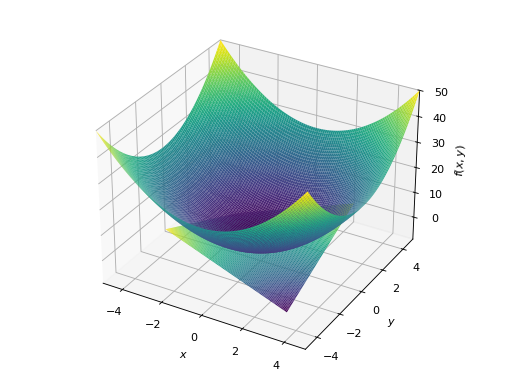Multiple plots.

>>> plot3d_parametric_line((cos(u), sin(u), u, (u, -5, 5)),
...     (sin(u), u**2, u, (u, -5, 5)))
Plot object containing:
: 3D parametric cartesian line: (cos(u), sin(u), u) for u over (-5.0, 5.0)
: 3D parametric cartesian line: (sin(u), u**2, u) for u over (-5.0, 5.0)


(png, hires.png, pdf)sympy.plotting.plot.plot3d_parametric_surface(*args, **kwargs)[source]

Plots a 3D parametric surface plot.

Usage

Single plot.

plot3d_parametric_surface(expr_x, expr_y, expr_z, range_u, range_v, **kwargs)

If the ranges is not specified, then a default range of (-10, 10) is used.

Multiple plots.

plot3d_parametric_surface((expr_x, expr_y, expr_z, range_u, range_v), ..., **kwargs)

Ranges have to be specified for every expression.

Default range may change in the future if a more advanced default range detection algorithm is implemented.

Arguments

expr_x: Expression representing the function along x.

expr_y: Expression representing the function along y.

expr_z: Expression representing the function along z.

range_u: (u, 0, 5), A 3-tuple denoting the range of the u variable.

range_v: (v, 0, 5), A 3-tuple denoting the range of the v variable.

Keyword Arguments

Arguments for ParametricSurfaceSeries class:

nb_of_points_u: int. The u range is sampled uniformly at nb_of_points_v of points

nb_of_points_y: int. The v range is sampled uniformly at nb_of_points_y of points

Aesthetics:

surface_color: Function which returns a float. Specifies the color for the surface of the plot. See sympy.plotting.Plot for more details.

If there are multiple plots, then the same series arguments are applied for all the plots. If you want to set these options separately, you can index the returned Plot object and set it.

Arguments for Plot class:

title : str. Title of the plot.

Examples

>>> from sympy import symbols, cos, sin
>>> from sympy.plotting import plot3d_parametric_surface
>>> u, v = symbols('u v')


Single plot.

>>> plot3d_parametric_surface(cos(u + v), sin(u - v), u - v,
...     (u, -5, 5), (v, -5, 5))
Plot object containing:
: parametric cartesian surface: (cos(u + v), sin(u - v), u - v) for u over (-5.0, 5.0) and v over (-5.0, 5.0)


(png, hires.png, pdf)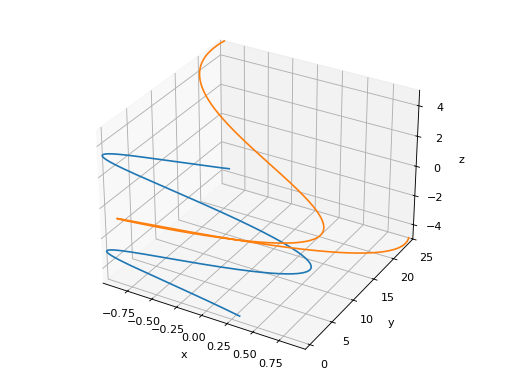sympy.plotting.plot_implicit.plot_implicit(expr, x_var=None, y_var=None, adaptive=True, depth=0, points=300, line_color='blue', show=True, **kwargs)[source]

A plot function to plot implicit equations / inequalities.

Arguments

• expr : The equation / inequality that is to be plotted.

• x_var (optional) : symbol to plot on x-axis or tuple giving symbol and range as (symbol, xmin, xmax)

• y_var (optional) : symbol to plot on y-axis or tuple giving symbol and range as (symbol, ymin, ymax)

If neither x_var nor y_var are given then the free symbols in the expression will be assigned in the order they are sorted.

The following keyword arguments can also be used:

• adaptive Boolean. The default value is set to True. It has to be

set to False if you want to use a mesh grid.

• depth integer. The depth of recursion for adaptive mesh grid.

Default value is 0. Takes value in the range (0, 4).

• points integer. The number of points if adaptive mesh grid is not

used. Default value is 300.

• show Boolean. Default value is True. If set to False, the plot will

not be shown. See Plot for further information.

• title string. The title for the plot.

• xlabel string. The label for the x-axis

• ylabel string. The label for the y-axis

Aesthetics options:

• line_color: float or string. Specifies the color for the plot.

See Plot to see how to set color for the plots. Default value is “Blue”

plot_implicit, by default, uses interval arithmetic to plot functions. If the expression cannot be plotted using interval arithmetic, it defaults to a generating a contour using a mesh grid of fixed number of points. By setting adaptive to False, you can force plot_implicit to use the mesh grid. The mesh grid method can be effective when adaptive plotting using interval arithmetic, fails to plot with small line width.

Examples

Plot expressions:

>>> from sympy import plot_implicit, cos, sin, symbols, Eq, And
>>> x, y = symbols('x y')


Without any ranges for the symbols in the expression

>>> p1 = plot_implicit(Eq(x**2 + y**2, 5))


With the range for the symbols

>>> p2 = plot_implicit(Eq(x**2 + y**2, 3),
...         (x, -3, 3), (y, -3, 3))


With depth of recursion as argument.

>>> p3 = plot_implicit(Eq(x**2 + y**2, 5),
...         (x, -4, 4), (y, -4, 4), depth = 2)


Using mesh grid and not using adaptive meshing.

>>> p4 = plot_implicit(Eq(x**2 + y**2, 5),
...         (x, -5, 5), (y, -2, 2), adaptive=False)


Using mesh grid with number of points as input.

>>> p5 = plot_implicit(Eq(x**2 + y**2, 5),
...         (x, -5, 5), (y, -2, 2),


Plotting regions.

>>> p6 = plot_implicit(y > x**2)


Plotting Using boolean conjunctions.

>>> p7 = plot_implicit(And(y > x, y > -x))


When plotting an expression with a single variable (y - 1, for example), specify the x or the y variable explicitly:

>>> p8 = plot_implicit(y - 1, y_var=y)
>>> p9 = plot_implicit(x - 1, x_var=x)


## PlotGrid Class¶

class sympy.plotting.plot.PlotGrid(nrows, ncolumns, *args, **kwargs)[source]

This class helps to plot subplots from already created sympy plots in a single figure.

Examples

>>> from sympy import symbols
>>> from sympy.plotting import plot, plot3d, PlotGrid
>>> x, y = symbols('x, y')
>>> p1 = plot(x, x**2, x**3, (x, -5, 5))
>>> p2 = plot((x**2, (x, -6, 6)), (x, (x, -5, 5)))
>>> p3 = plot(x**3, (x, -5, 5))
>>> p4 = plot3d(x*y, (x, -5, 5), (y, -5, 5))(png, hires.png, pdf)(png, hires.png, pdf)(png, hires.png, pdf)(png, hires.png, pdf)

Plotting vertically in a single line:

>>> PlotGrid(2, 1 , p1, p2)
PlotGrid object containing:
Plot:Plot object containing:
: cartesian line: x for x over (-5.0, 5.0)
: cartesian line: x**2 for x over (-5.0, 5.0)
: cartesian line: x**3 for x over (-5.0, 5.0)
Plot:Plot object containing:
: cartesian line: x**2 for x over (-6.0, 6.0)
: cartesian line: x for x over (-5.0, 5.0)


(png, hires.png, pdf)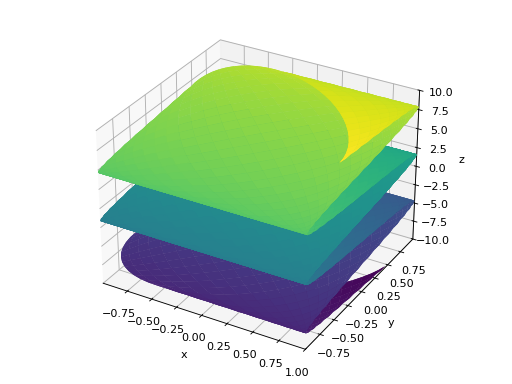Plotting horizontally in a single line:

>>> PlotGrid(1, 3 , p2, p3, p4)
PlotGrid object containing:
Plot:Plot object containing:
: cartesian line: x**2 for x over (-6.0, 6.0)
: cartesian line: x for x over (-5.0, 5.0)
Plot:Plot object containing:
: cartesian line: x**3 for x over (-5.0, 5.0)
Plot:Plot object containing:
: cartesian surface: x*y for x over (-5.0, 5.0) and y over (-5.0, 5.0)


(png, hires.png, pdf)Plotting in a grid form:

>>> PlotGrid(2, 2, p1, p2 ,p3, p4)
PlotGrid object containing:
Plot:Plot object containing:
: cartesian line: x for x over (-5.0, 5.0)
: cartesian line: x**2 for x over (-5.0, 5.0)
: cartesian line: x**3 for x over (-5.0, 5.0)
Plot:Plot object containing:
: cartesian line: x**2 for x over (-6.0, 6.0)
: cartesian line: x for x over (-5.0, 5.0)
Plot:Plot object containing:
: cartesian line: x**3 for x over (-5.0, 5.0)
Plot:Plot object containing:
: cartesian surface: x*y for x over (-5.0, 5.0) and y over (-5.0, 5.0)


(png, hires.png, pdf)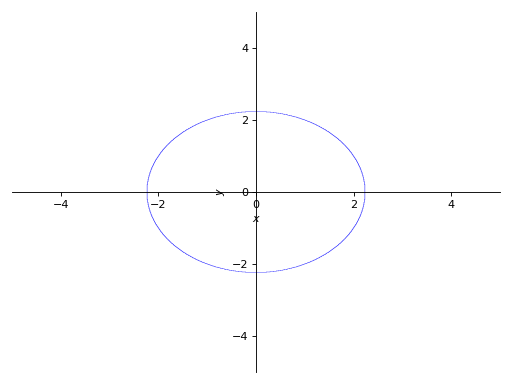## Series Classes¶

class sympy.plotting.plot.BaseSeries[source]

Base class for the data objects containing stuff to be plotted.

The backend should check if it supports the data series that it’s given. (eg TextBackend supports only LineOver1DRange). It’s the backend responsibility to know how to use the class of data series that it’s given.

Some data series classes are grouped (using a class attribute like is_2Dline) according to the api they present (based only on convention). The backend is not obliged to use that api (eg. The LineOver1DRange belongs to the is_2Dline group and presents the get_points method, but the TextBackend does not use the get_points method).

class sympy.plotting.plot.Line2DBaseSeries[source]

A base class for 2D lines.

• adding the label, steps and only_integers options

• making is_2Dline true

• defining get_segments and get_color_array

class sympy.plotting.plot.LineOver1DRangeSeries(expr, var_start_end, **kwargs)[source]

Representation for a line consisting of a SymPy expression over a range.

get_segments()[source]

The adaptive sampling is done by recursively checking if three points are almost collinear. If they are not collinear, then more points are added between those points.

References

R601

Adaptive polygonal approximation of parametric curves, Luiz Henrique de Figueiredo.

class sympy.plotting.plot.Parametric2DLineSeries(expr_x, expr_y, var_start_end, **kwargs)[source]

Representation for a line consisting of two parametric sympy expressions over a range.

get_segments()[source]

The adaptive sampling is done by recursively checking if three points are almost collinear. If they are not collinear, then more points are added between those points.

References

 Adaptive polygonal approximation of parametric curves,

Luiz Henrique de Figueiredo.

class sympy.plotting.plot.Line3DBaseSeries[source]

A base class for 3D lines.

Most of the stuff is derived from Line2DBaseSeries.

class sympy.plotting.plot.Parametric3DLineSeries(expr_x, expr_y, expr_z, var_start_end, **kwargs)[source]

Representation for a 3D line consisting of two parametric sympy expressions and a range.

class sympy.plotting.plot.SurfaceBaseSeries[source]

A base class for 3D surfaces.

class sympy.plotting.plot.SurfaceOver2DRangeSeries(expr, var_start_end_x, var_start_end_y, **kwargs)[source]

Representation for a 3D surface consisting of a sympy expression and 2D range.

class sympy.plotting.plot.ParametricSurfaceSeries(expr_x, expr_y, expr_z, var_start_end_u, var_start_end_v, **kwargs)[source]

Representation for a 3D surface consisting of three parametric sympy expressions and a range.

class sympy.plotting.plot_implicit.ImplicitSeries(expr, var_start_end_x, var_start_end_y, has_equality, use_interval_math, depth, nb_of_points, line_color)[source]

Representation for Implicit plot

## Pyglet Plotting¶

This is the documentation for the old plotting module that uses pyglet. This module has some limitations and is not actively developed anymore. For an alternative you can look at the new plotting module.

The pyglet plotting module can do nice 2D and 3D plots that can be controlled by console commands as well as keyboard and mouse, with the only dependency being pyglet.

Here is the simplest usage:

>>> from sympy import var, Plot
>>> var('x y z')
>>> Plot(x*y**3-y*x**3)


To see lots of plotting examples, see examples/pyglet_plotting.py and try running it in interactive mode (python -i plotting.py):

\$ python -i examples/pyglet_plotting.py


And type for instance example(7) or example(11).

## Plot Window Controls¶

Camera

Keys

Sensitivity Modifier

SHIFT

Zoom

R and F, Page Up and Down, Numpad + and -

Rotate View X,Y axis

Rotate View Z axis

Q and E, Numpad 7 and 9

Rotate Ordinate Z axis

Z and C, Numpad 1 and 3

View XY

F1

View XZ

F2

View YZ

F3

View Perspective

F4

Reset

Axes

Keys

Toggle Visible

F5

Toggle Colors

F6

Window

Keys

Close

ESCAPE

Screenshot

F8

The mouse can be used to rotate, zoom, and translate by dragging the left, middle, and right mouse buttons respectively.

## Coordinate Modes¶

Plot supports several curvilinear coordinate modes, and they are independent for each plotted function. You can specify a coordinate mode explicitly with the ‘mode’ named argument, but it can be automatically determined for cartesian or parametric plots, and therefore must only be specified for polar, cylindrical, and spherical modes.

Specifically, Plot(function arguments) and Plot.__setitem__(i, function arguments) (accessed using array-index syntax on the Plot instance) will interpret your arguments as a cartesian plot if you provide one function and a parametric plot if you provide two or three functions. Similarly, the arguments will be interpreted as a curve is one variable is used, and a surface if two are used.

Supported mode names by number of variables:

• 1 (curves): parametric, cartesian, polar

• 2 (surfaces): parametric, cartesian, cylindrical, spherical

>>> Plot(1, 'mode=spherical; color=zfade4')


Note that function parameters are given as option strings of the form “key1=value1; key2 = value2” (spaces are truncated). Keyword arguments given directly to plot apply to the plot itself.

## Specifying Intervals for Variables¶

The basic format for variable intervals is [var, min, max, steps]. However, the syntax is quite flexible, and arguments not specified are taken from the defaults for the current coordinate mode:

>>> Plot(x**2) # implies [x,-5,5,100]
>>> Plot(x**2, [], []) # [x,-1,1,40], [y,-1,1,40]
>>> Plot(x**2-y**2, , ) # [x,-1,1,100], [y,-1,1,100]
>>> Plot(x**2, [x,-13,13,100])
>>> Plot(x**2, [-13,13]) # [x,-13,13,100]
>>> Plot(x**2, [x,-13,13]) # [x,-13,13,100]
>>> Plot(1*x, [], [x], 'mode=cylindrical') # [unbound_theta,0,2*Pi,40], [x,-1,1,20]


## Using the Interactive Interface¶

>>> p = Plot(visible=False)
>>> f = x**2
>>> p = f
>>> p = f.diff(x)
>>> p = f.diff(x).diff(x)
>>> p
: x**2, 'mode=cartesian'
: 2*x, 'mode=cartesian'
: 2, 'mode=cartesian'
>>> p.show()
>>> p.clear()
>>> p
<blank plot>
>>> p =  x**2+y**2
>>> p.style = 'solid'
>>> p = -x**2-y**2
>>> p.style = 'wireframe'
>>> p.color = z, (0.4,0.4,0.9), (0.9,0.4,0.4)
>>> p.style = 'both'
>>> p.style = 'both'
>>> p.close()


## Using Custom Color Functions¶

The following code plots a saddle and color it by the magnitude of its gradient:

>>> fz = x**2-y**2
>>> Fx, Fy, Fz = fz.diff(x), fz.diff(y), 0
>>> p = fz, 'style=solid'
>>> p.color = (Fx**2 + Fy**2 + Fz**2)**(0.5)


The coloring algorithm works like this:

1. Evaluate the color function(s) across the curve or surface.

2. Find the minimum and maximum value of each component.

3. Scale each component to the color gradient.

When not specified explicitly, the default color gradient is f(0.0)=(0.4,0.4,0.4) -> f(1.0)=(0.9,0.9,0.9). In our case, everything is gray-scale because we have applied the default color gradient uniformly for each color component. When defining a color scheme in this way, you might want to supply a color gradient as well:

>>> p.color = (Fx**2 + Fy**2 + Fz**2)**(0.5), (0.1,0.1,0.9), (0.9,0.1,0.1)


Here’s a color gradient with four steps:

>>> gradient = [ 0.0, (0.1,0.1,0.9), 0.3, (0.1,0.9,0.1),
...              0.7, (0.9,0.9,0.1), 1.0, (1.0,0.0,0.0) ]
>>> p.color = (Fx**2 + Fy**2 + Fz**2)**(0.5), gradient


The other way to specify a color scheme is to give a separate function for each component r, g, b. With this syntax, the default color scheme is defined:

>>> p.color = z,y,x, (0.4,0.4,0.4), (0.9,0.9,0.9)


This maps z->red, y->green, and x->blue. In some cases, you might prefer to use the following alternative syntax:

>>> p.color = z,(0.4,0.9), y,(0.4,0.9), x,(0.4,0.9)


You can still use multi-step gradients with three-function color schemes.

## Plotting Geometric Entities¶

The plotting module is capable of plotting some 2D geometric entities like line, circle and ellipse. The following example plots a circle and a tangent line at a random point on the ellipse.

In : p = Plot(axes='label_axes=True')

In : c = Circle(Point(0,0), 1)

In : t = c.tangent_line(c.random_point())

In : p = c

In : p = t


Plotting polygons (Polygon, RegularPolygon, Triangle) are not supported directly. However a polygon can be plotted through a loop as follows.

In : p = Plot(axes='label_axes=True')

In : t = RegularPolygon(Point(0,0), 1, 5)

In : for i in range(len(t.sides)):
....:    p[i] = t.sides[i]


## Plotting with ASCII art¶

sympy.plotting.textplot.textplot(expr, a, b, W=55, H=18)[source]

Print a crude ASCII art plot of the SymPy expression ‘expr’ (which should contain a single symbol, e.g. x or something else) over the interval [a, b].

Examples

>>> from sympy import Symbol, sin
>>> from sympy.plotting import textplot
>>> t = Symbol('t')
>>> textplot(sin(t)*t, 0, 15)
14.1605 |                                                   ...
|                                                      .
|                                                  .
|                                                 .     .
|                             ..
|                            /  ..               .
|                           /     .
|                          /
2.30284 | ------...---------------/--------.------------.--------
|   ....   ...           /
| ..          \         /           .          .
|              ..      /             .
|                ..   /                       .
|                  ...                .
|                                            .
|                                      .
|                                       \   .
-11.037 |                                        ...
0                          7.5                        15